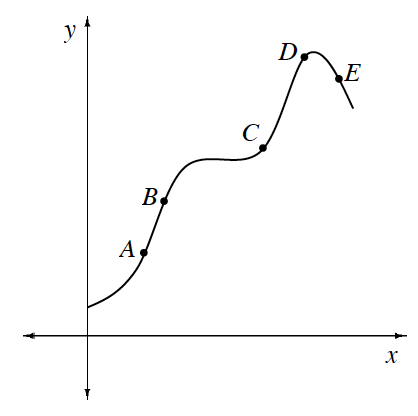### Home > APCALC > Chapter 6 > Lesson 6.4.2 > Problem6-137

6-137.

While answering the questions below, refer to the graph at right. Assume the axes are scaled equally. .

1. Between which pair of consecutive points is the average rate of change the greatest?

Average rate of change (AROC) can be seen on a graph as the slope of the secant line between two points.

2. At which point is the instantaneous rate of change closest to $1$?

Instantaneous rate of change (IROC) can be seen on a graph as the slope of the tangent line at a specific point.

3. Which is greater: the average rate of change between $B$ and $C$ or the instantaneous rate of change at $C$?

Which is greater: the slope of the secant line or the slope of the tangent line?

4. Which is greater: the average rate of change between $C$ and $D$ or the average rate of change between $C$ and $E$?

Refer to the previous hints.

Use the eTool below to visualize the problem.
Click the link at right for the full version of the eTool: Calc 6-137 HW eTool